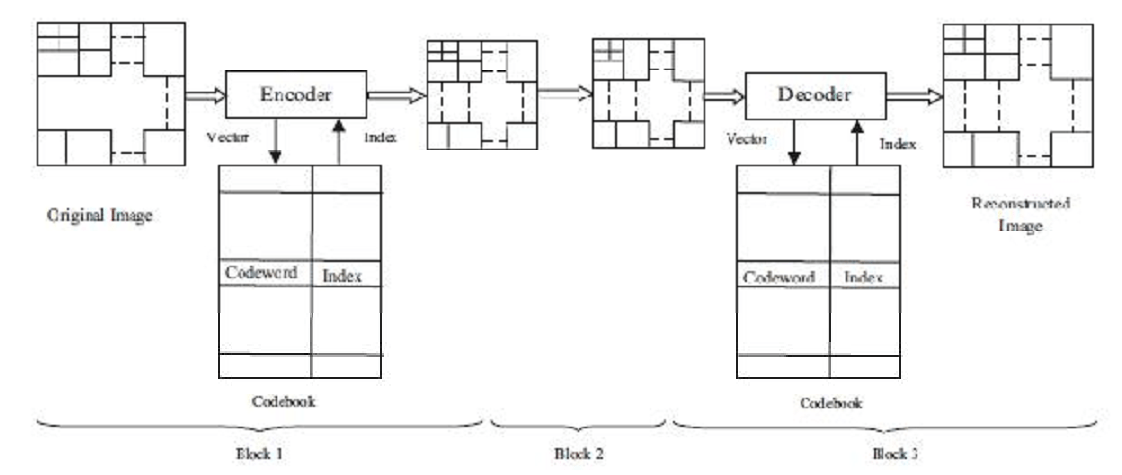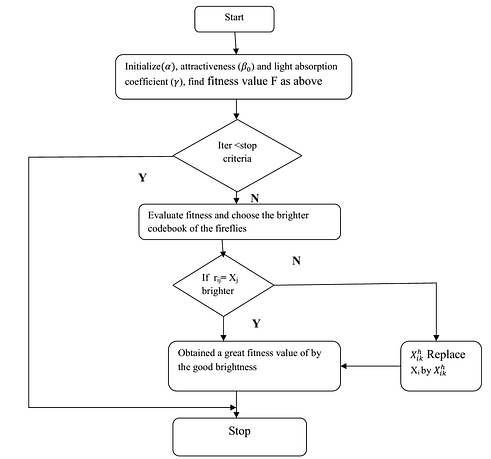# Image Compression by optimized vector Quantization

\$200.00

Total downloads: 5

The image compression is mandatory to transfer the image over communication medium to save the bandwidth. This topic is discussing the lossy image compression algorithm which is using optimized vector qunatization method.

This Product includes:

• MATLAB code
• Relevant papers
• Explanation doc

Note:We dont claim the documentation file to be plagiarism free and neither support to copy this code for your academic submission. This is to ease your pain to start writing code from scratch. We suggest to modify the code for your work.

## Description

The vector Quantization used for the image compression. The vector quantization carried out by three steps encoder, channel and decoder. The basic arrangement of vector quantization is shown in the figure where blocks represent the overall process for image compression system. Vector quantization compresses the size of the image by using the optimization algorithm. We can use FFA and ALO algorithm for the image compression system. In vector quantization the input is given as a image after that the codeword and index is generated for that image then transmitted through the channel. All the information is in coding form. The information is received by the decoder which decodes the image parameter and obtained the actual image.Figure: block diagram of vector quantization process

There are three blocks in the figure which perform individual operation for the image compression.

• Block 1- The block 1 basically performs the encoder function like generation of image vector, codebook generation and indexing. The codebook is generally efficient so algorithm is also efficient.
• Block 2- The block 2 work as a channel through which the index number are transmitted to the receiver.
• Block 3- The block 3 is the decoder it includes index table, codebook and reconstruct images.

LBG Vector Quantization Algorithm

It is the most commonly method used for the vector quantization. The basic steps of this algorithm are:

Step 1. Begin with initial codebook C1 of size N. Let the iteration counter be m =1 and the initial distortion D1 =1.

Step2: Using codebook Cm = {Yi}, partition the training set into cluster sets Ri using the nearest neighbor condition.

Step 3: Once the mapping of all the input vectors to the initial code vectors is made, compute the centroids of the partition region found in step 2. This gives an improved codebook Cm+1.

Step 4: Calculate the average distortion Dm+1. If Dm _ Dm+1<T then stops, otherwise m= m+ 1 and repeat step 2 to step 4.

The various methods are used for the image compression system optimization. We used firefly algorithm for vector quantization for LBG scheme.

FFA LBG vector quantization algorithm

The basic principle of firefly algorithm is flashing pattern and characteristics of fireflies. In this algorithm we are assuming that brightness of a firefly is equal to objective function value. The lower intensity firefly (lower fitness value) moves toward brighter firefly (higher fitness value).

The steps for the firefly algorithm for vector quantization are:

Step 1: Run the LBG algorithm and assign its outcome as brighter codebook.

Step 2: Initialize alpha, attractiveness () and light absorption coefficient () parameters. Initialize rest codebooks with random numbers.

Step 3: Find fitness value of each codebook by Equation belowwhere Xi is the ith input image vector and Cj is jth codeword of size Nb in a codebook of size Nc and uij is 1 if Xi is in the jth cluster, otherwise zero.

Step 4: Randomly select a codebook and record its fitness value. If there is a brighter codebook, then it moves toward the brighter codebook (highest fitness value) based on EquationsHere Xi is randomly selected codebook, Xj is brighter Codebook,where u is a random number between 0 and 1, k = 1,2,. . . , Nc, h= 1,2,. . . ,L.

Step 5: If no firefly fitness value is better than the selected firefly then it moves randomly in search space according to Equation (5)Step 6: Repeat step 3 to step 5 until one of the termination criteria is reached.

The Flow chart is shown asFlow chart for Image compression

## Reviews

There are no reviews yet.

Only logged in customers who have purchased this product may leave a review.